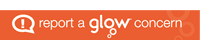# BGE Mathematics

### Numbers and number processes

Whole numbers Number problems using +/-/÷/x

Place value

Multiplying/dividing by 10, 100, 1000

Estimating & rounding with whole numbers, tens and up to 2 decimal places

Powers and square roots

BODMAS

Using a calculator

Negative numbers

### Angles

Naming angles

Classifying angles

Drawing angles.

Using angle facts to calculate their sizes

### Algebra

Continuing sequences

Square + triangular numbers

Simple linear patterns

Expressions – collecting like terms

Equations

Forming expressions + equations

Inequalities

### Decimals, Fractions + Percentages

Fractions/Decimals Percentages relationship

Calculations involving Fractions, Decimals and Percentages

Convert between Whole Number and mixed Fractions

### Measurement – 2D shapes

Units of length conversions

Perimeters including compound shapes

Areas of – rectangles, composite shapes and

Right-angled triangles

Drawing 2D shapes

Triangles

### Data & Analysis and Probability

Means, Medians, Mode and Range

Frequency tables and Mean from frequency tables

Line Graphs, Bar Graphs

Stem and Leaf graphs

Scatter graphs and Line of Best fit

Probability and calculating probabilities

Surveys and bias

### Time

12 – 24 hour clock conversions

Time intervals

Timetables

Time zones

Speed Distance and Time calculations

### Symmetry and Properties of 2D shapes

Identifying lines of symmetry in 2D shapes.

Complete diagrams and patterns so that they are symmetrical.

Use symmetry to identify and then use the properties of a range of 2D shapes.

Rotational symmetry

### Nets and Volumes of 3D Shapes

Drawing Nets of 3D shapes

Working out surface areas of 3D shapes

Working out volumes of 3D composite shapes

### Scale Drawing

Interpreting scales

Making scale drawings (including with protractors)

Compass points and Three Figure Bearings

Scale enlargement and reduction

### Ratio and Proportion

Understanding Ratios

Simplifying Ratios

Ratio Calculations

Sharing out using a ratio

Direct Proportion

Direct Proportion graphs

Inverse Proportion

### Money and Money Products

Money Products

Money in real life/research into real money situations, buying, selling etc.

Managing Money

### Circles

The names for different parts of circle

The link between Circumference and Diameter and using C= 3.14*D

Work with semi circles and quarter circles.

The link between area and radius and using A = 3.14 r^2

### Multiple, Primes and Factors

Multiples (including Least Common Multiple)

Factors (including Highest Common Factor)

Prime Numbers

Removing a Common Factor

Simple Factorisation

## Inspiring Achievement